# Package ‘odr’

#### 2020-03-13

The costs of sampling each additional unit in multilevel experimental studies vary across levels of hierarchy and treatment conditions due to the hierarchical sampling and the delivery of treatment. This package is a tool to optimize the designs of multilevel experimental studies such that the variances of treatment effects are minimized under a fixed budget and cost structure, or the budget is minimized to achieve same level design precision or statistical power. The optimal sample allocation or optimal design parameters include

• the optimal sample sizes at each of the levels except the top level because the top-level sample size will be determined by total budget or power once all other parameters are decided
• proportion of units to be assigned to the treatment condition at the level of randomization

This package includes three categorical of functions and they are

• od function: This function calculates optimal sample allocation parameters with and without constraint(s). For each type of multilevel experimental studies, there is an additional number (and/or additional letter) to be added to the general function name. For example, the function for two-level cluster randomized trials is od.2, the function for two-level multisite randomized trials is od.2m, the function for single-level experiments assessing mediation effects is od.1.m.

• power function: This function performs power analyses with and without accommodating cost structures of sampling. For power analysis accommodating cost structures, depending on which one parameter is left, the function calculates required budget (and sample size), statistical power, and minimum detectable effect size. For conventional power analysis without accommodating cost structures of sampling, this function calculates required sample size, statistical power, and minimum detectable effect size. For each type of multilevel experimental studies, there is an additional number (and/or additional letter) to be added to the general function name. For example, the function for two-level cluster randomized trials is power.2, the function for two-level multisite randomized trials is power.2m, the function for single-level experiments assessing mediation effects is power.1.m.

• re function: This function calculates relative efficiency values between two designs. An alternative name for this function is rpe that stands for “relative precision and efficiency”.

## 1. Function od

Given cost structure (i.e., the costs of sampling each unit at different levels and treatment conditions), this function solves the optimal sample allocation with and without constraints.

To solve the optimal sample allocation of a two-level cluster-randomized trial, we need the following information

• icc: intraclass correlation coefficient
• r12: the proportion of level-one outcome variance explained by covariates
• r22: the proportion of level-two outcome variance explained by covariates
• c1: the cost of sampling each additional level-one unit in the control condition
• c2: the cost of sampling each additional level-two unit in the control condition
• c1t: the cost of sampling each additional level-one unit in the experimental condition
• c2t: the cost of sampling each additional level-two unit in the experimental condition
• m: a total fixed budget used to plot the variance curves, default value is the cost of sampling 60 level-two units across treatment conditions.

### 1.1 Examples

library(odr)
 # unconstrained optimal design
myod1 <- od.2(icc = 0.2, r12 = 0.5, r22 = 0.5, c1 = 1, c2 = 5, c1t = 1, c2t = 50,
varlim = c(0.01, 0.02))
## The optimal level-1 sample size per level-2 unit (n) is 8.878572.
## The optimal proportion of level-2 units in treatment (p) is 0.326828.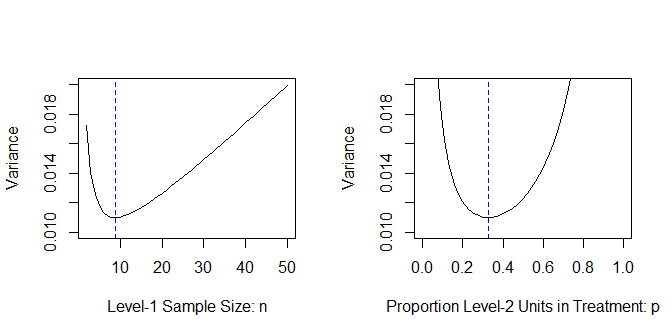# The function by default prints messages of output and plots the variance curves; one can turn off message and specify one or no plot.
# myod1$out # output; # myod1$par # parameters used in the calculation.
 # constrained optimal design with n = 20
myod2 <- od.2(icc = 0.2, r12 = 0.5, r22 = 0.5, c1 = 1, c2 = 5, c1t = 1, c2t = 50,
plot.by = list(p = "p"), n = 20, varlim = c(0.005, 0.030))
## The constrained level-1 sample size per level-2 unit (n) is 20.
## The optimal proportion of level-2 units in treatment (p) is 0.3740667.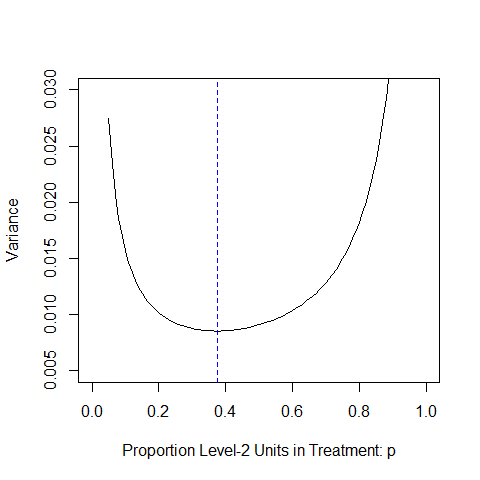# myod2$out # output # myod2$par # parameters used in the calculation.
 # constrained optimal design with p = 0.5
myod3 <- od.2(icc = 0.2, r12 = 0.5, r22 = 0.5, c1 = 1, c2 = 5, c1t = 1, c2t = 50,
p = 0.5, varlim = c(0.005, 0.020))
## The optimal level-1 sample size per level-2 unit (n) is 10.48809.
## The constrained proportion of level-2 units in treatment (p) is 0.5.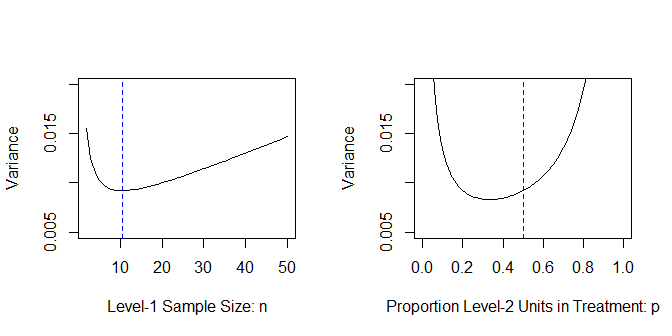# myod3$out # output; # myod3$par # parameters used in the calculation.
 # constrained n and p, no calculation performed
myod4 <- od.2(icc = 0.2, r12 = 0.5, r22 = 0.5, c1 = 1, c2 = 5, c1t = 1, c2t = 50,
plots = FALSE, n = 20, p = 0.5, varlim = c(0.005, 0.025))
## ===============================
## Both p and n are constrained, there is no calculation from other parameters.
## ===============================
## The constrained level-1 sample size per level-2 unit (n) is 20.
## The constrained proportion of level-2 units in treatment (p) is 0.5.

### 1.2 Examples for other types of trials

Please see examples in corresponding functions by uncommenting below lines.

# ?od.1
# ?od.3
# ?od.4
# ?od.2m
# ?od.3m
# ?od.4m

## 2. Function power

This function by default can perform power analyses accommodating cost structures (i.e., cost.model = TRUE), one of ‘power’, ‘m’, and ‘d’ must be NULL. For example, if ‘power’ is NULL, the function calculates statistical power under a fixed budget and cost structure; if ‘d’ is NULL, the function calculates minimum detectable effect size (i.e., d) under a fixed budget and desired power level; if ‘m’ is NULL, the function calculate required budget (and required sample size) to achieve desired power level to detect a treatment effect.

This function also can conduct conventional power analysis or power analysis wihout accommodating cost structures by specifying cost.model = FALSE, the conventional power analyses include statistical power calculation, minimum detectable effect size calculation, and required sample size calculation.

### 2.1 Examples of power analyses accommodating cost structures (cost.model = TRUE)

###### Required budget for desired power
• Required budget calculation
mym <- power.2(expr = myod1, d = 0.3, q = 1, power = 0.8)
# mym$out # m =1702, J = 59 • Effects on required budget to maintain same level power when designs depart from the optimal one figure <- par(mfrow = c(1, 2)) budget <- NULL nrange <- c(2:50) for (n in nrange) budget <- c(budget, power.2(expr = myod1, constraint = list (n = n), d = 0.3, q = 1, power = 0.8)$out$m) plot(nrange, budget, type = "l", lty = 1, xlim = c(0, 50), ylim = c(1500, 3500), xlab = "Level-1 sample size: n", ylab = "Budget", main = "", col = "black") abline(v = 9, lty = 2, col = "Blue") budget <- NULL prange <- seq(0.05, 0.95, by = 0.005) for (p in prange) budget <- c(budget, power.2(expr = myod1, constraint = list (p = p), d = 0.3, q = 1, power = 0.8)$out$m) plot(prange, budget, type = "l", lty = 1, xlim = c(0, 1), ylim = c(1500, 7000), xlab = "Porportion groups in treatment: p", ylab = "Budget", main = "", col = "black") abline(v = 0.33, lty = 2, col = "Blue")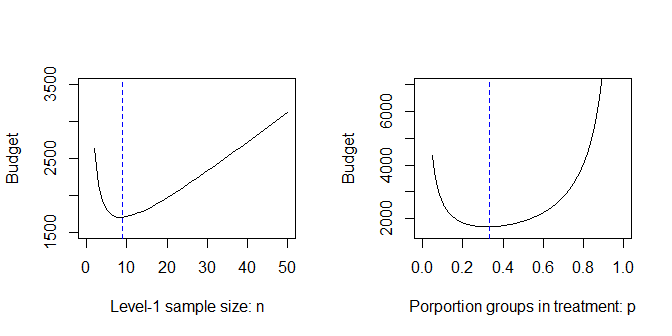par(figure) ###### Statistical power under a fixed budget • Power calculation mypower <- power.2(expr = myod1, q = 1, d = 0.3, m = 1702) # mypower$out  # power = 0.80
• Effects on power under same budget when designs depart from the optimal one
figure <- par(mfrow = c (1, 2))
pwr <- NULL
nrange <- c(2:50)
for (n in nrange)
pwr <- c(pwr, power.2(expr = myod1, constraint = list (n = n), d = 0.3, q = 1, m = 1702)$out) plot(nrange, pwr, type = "l", lty = 1, xlim = c(0, 50), ylim = c(0.4, 0.9), xlab = "Level-1 sample size: n", ylab = "Power", main = "", col = "black") abline(v = 9, lty = 2, col = "Blue") pwr <- NULL prange <- seq(0.05, 0.95, by = 0.005) for (p in prange) pwr <- c(pwr, power.2(expr = myod1, constraint = list (p = p), d = 0.3, q = 1, m = 1702)$out)
plot(prange, pwr, type = "l", lty = 1, xlim = c(0, 1), ylim = c(0.1, 0.9),
xlab = "Porportion groups in treatment: p", ylab = "Power",  main = "", col = "black")
abline(v = 0.33, lty = 2, col = "Blue")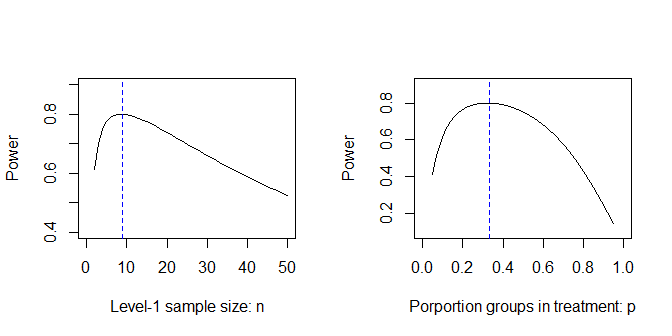par(figure)
###### Minimum detectable effect size under a fixed budget
• minimum detectable effect size calculation
mymdes <- power.2(expr = myod1, q = 1, power = 0.80, m = 1702)
# above experssion takes parameters and outputs from od.2 function. Equivalently, each parameter can be explicitly specified.
# mym <- power.2(icc = 0.2, r12 = 0.5, r22 = 0.5, c1 = 1, c2 = 5, c1t = 1, c2t = 50,
#                     n = 9, p = 0.33, d = 0.3, q = 1, power = 0.8)
# mymdes$out # d = 0.30 • Effects on minimum detectable effect size under same budget when designs depart from the optimal one figure <- par(mfrow = c (1, 2)) MDES <- NULL nrange <- c(2:50) for (n in nrange) MDES <- c(MDES, power.2(expr = myod1, constraint = list (n = n), power = 0.8, q = 1, m = 1702)$out)
plot(nrange, MDES, type = "l", lty = 1, xlim = c(0, 50), ylim = c(0.3, 0.8),
xlab = "Level-1 sample size: n", ylab = "MDES", main = "", col = "black")
abline(v = 9, lty = 2, col = "Blue")

MDES <- NULL
prange <- seq(0.05, 0.95, by = 0.005)
for (p in prange)
MDES <- c(MDES, power.2(expr = myod1, constraint = list (p = p), power = 0.8, q = 1, m = 1702)$out) plot(prange, MDES, type = "l", lty = 1, xlim = c(0, 1), ylim = c(0.3, 0.8), xlab = "Porportion groups in treatment: p", ylab = "MDES", main = "", col = "black") abline(v = 0.33, lty = 2, col = "Blue")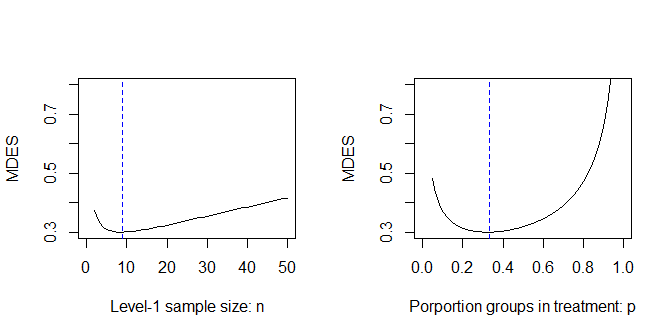par(figure) ### 2.2 Examples of conventional power analyses (cost.model = FALSE) # Required level-2 sample size calculation myJ <- power.2(cost.model = FALSE, expr = myod1, d = 0.3, q = 1, power = 0.8) # above experssion takes parameters and outputs from od.2 function. Equivalently, each parameter can be explicitly specified. # myJ <- power.2(icc = 0.2, r12 = 0.5, r22 = 0.5, # cost.model = FALSE, n = 9, p = 0.33, d = 0.3, q = 1, power = 0.8) myJ$out  # J = 59
## $J ##  58.99295 # Power calculation mypower1 <- power.2(cost.model = FALSE, expr = myod1, J = 59, d = 0.3, q = 1) mypower1$out  # power = 0.80
## $power ##  0.8000486 # Minimum detectable effect size calculation mymdes1 <- power.2(cost.model = FALSE, expr = myod1, J = 59, power = 0.8, q = 1) mymdes1$out  # d = 0.30
## $d ##  0.2999819 ### 2.3 Examples of conventional power curves figure <- par(mfrow = c (1, 2)) pwr <- NULL mrange <- c(300:3000) for (m in mrange) pwr <- c(pwr, power.2(expr = myod1, d = 0.3, q = 1, m = m)$out)
plot(mrange, pwr, type = "l", lty = 1, xlim = c(300, 3000), ylim = c(0, 1),
xlab = "Budget", ylab = "Power", main = "", col = "black")
abline(v = 1702, lty = 2, col = "Blue")

pwr <- NULL
Jrange <- c(4:100)
for (J in Jrange)
pwr <- c(pwr, power.2(expr = myod1, cost.model = FALSE, d = 0.3, q = 1, J = J)$out) plot(Jrange, pwr, type = "l", lty = 1, xlim = c(4, 100), ylim = c(0, 1), xlab = "Level-2 sample size: J", ylab = "Power", main = "", col = "black") abline(v = 59, lty = 2, col = "Blue")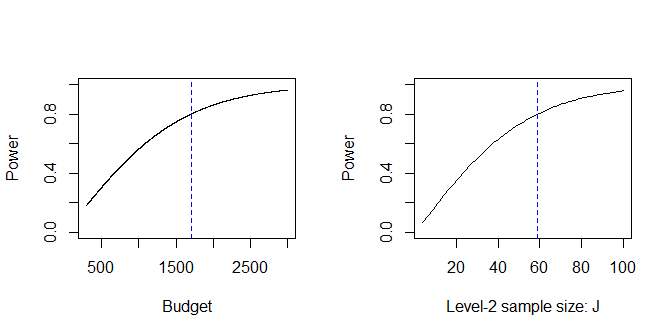par(figure) ### 2.4 Examples for other types of trials Please see examples in corresponding functions by uncommenting below lines. # ?power.1 # ?power.3 # ?power.4 # ?power.2m # ?power.3m # ?power.4m ## 3. Function re Calculate the relative efficiency (RE) of two designs, this function uses the returns from od function ### 3.1 Examples Based on above examples in od functions, calculate the relative efficiency # relative efficiency (RE) of a constrained design comparing with the optimal design myre <- re(od = myod1, subod= myod2) ## The relative efficiency (RE) of the two two-level CRTs is 0.8790305. myre$re # get the output (i.e., RE = 0.88)
##  0.8790305
# relative efficiency (RE) of a constrained design comparing with the unconstrained optimal one
myre <- re(od = myod1, subod= myod3)
## The relative efficiency (RE) of the two two-level CRTs is 0.8975086.
# relative efficiency (RE) of a constrained design comparing with the unconstrained optimal one
myre <- re(od = myod1, subod= myod4)
## The relative efficiency (RE) of the two two-level CRTs is 0.8266527.

### 3.2 Examples for other types of trials

For additional examples, please see example sections in corresponding od functions by uncommenting below lines.

# ?od.1
# ?od.2
# ?od.3
# ?od.4
# ?od.2m
# ?od.3m
# ?od.4m